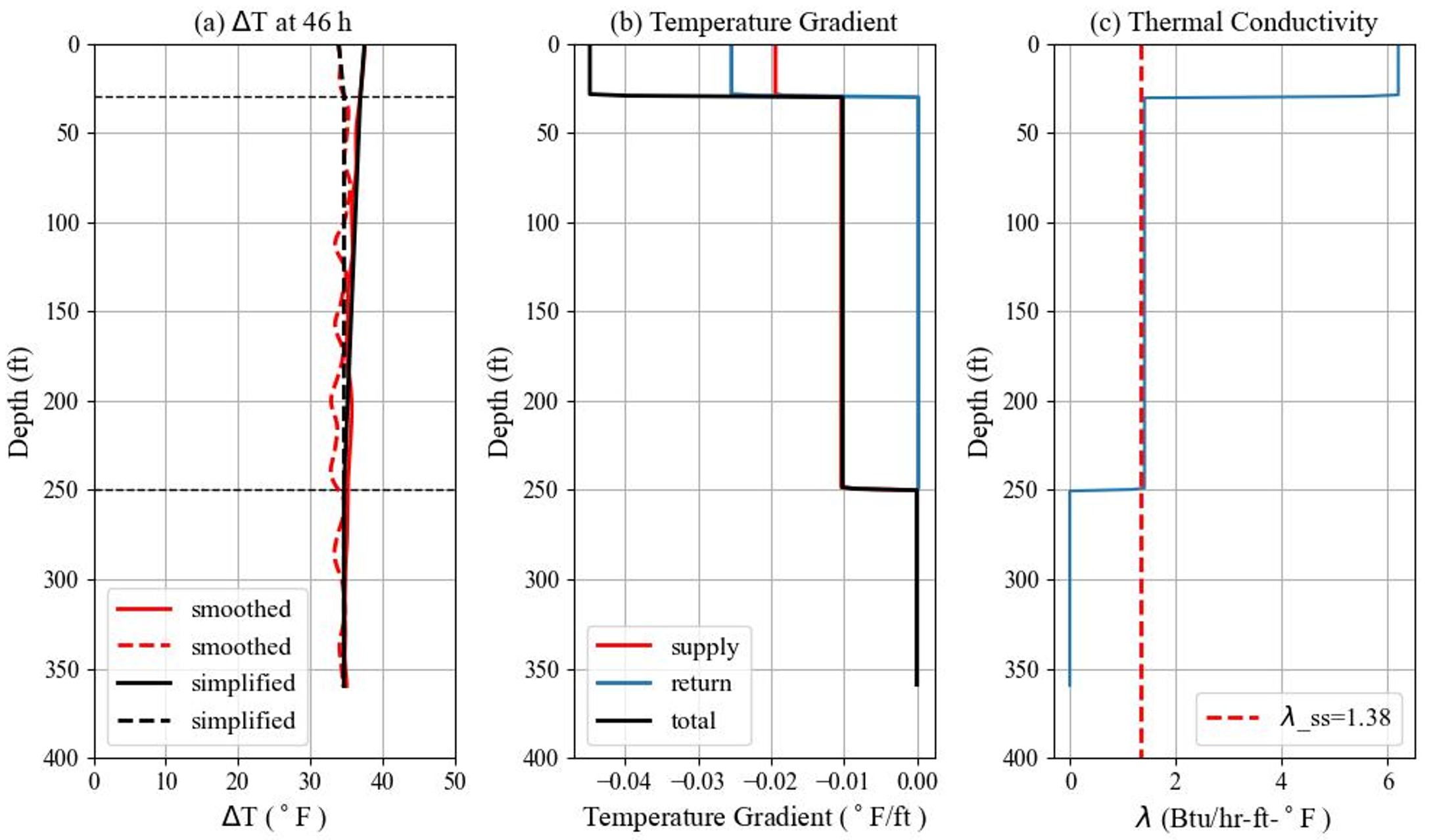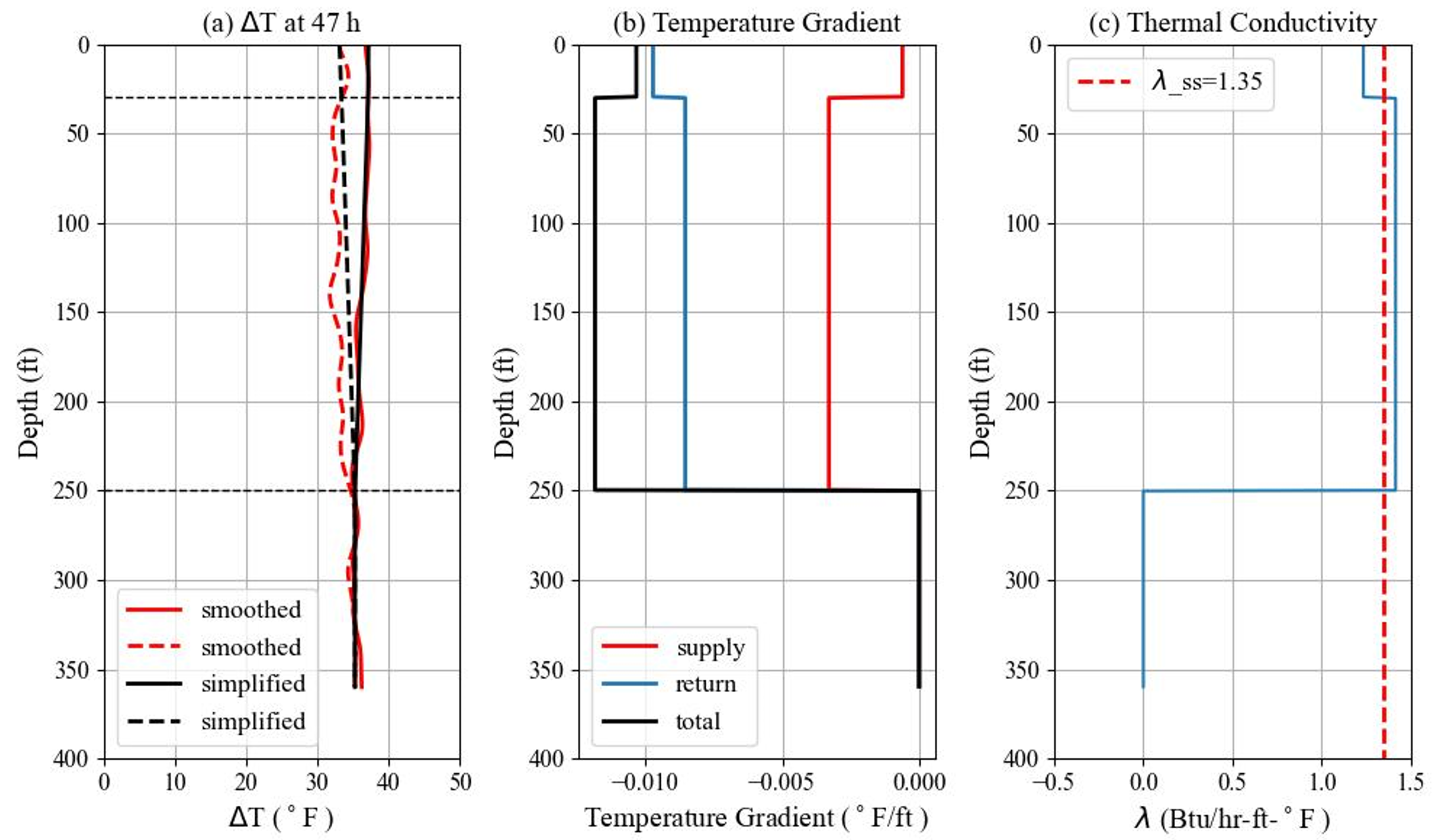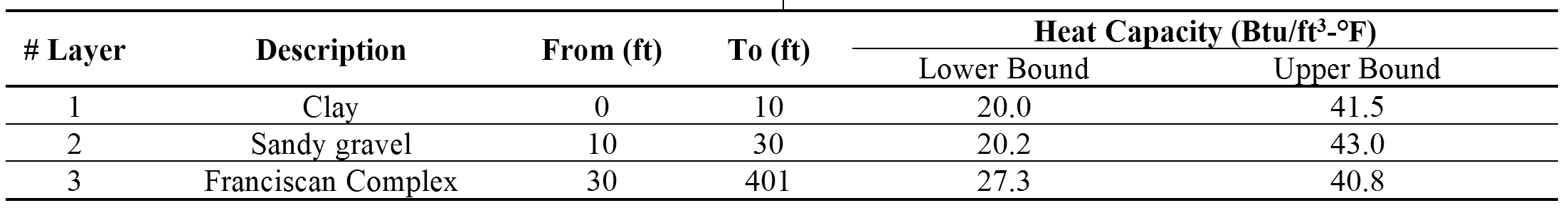# Estimate of Geothermal Property Profile

The subsurface was divided into three main layers, and the analytical method developed by McDaniel et al. (2018) was applied as a fast method to estimate the thermal conductivity profile. This method assumes the radial temperature gradients from the U-tube to the subsurface are constant and uniform at the steady state.

Results of two DTRTs are shown in Figure 1 and 2. In the first test, λ for the sediment above 30 ft is 6.2 Btu/hr-ft-℉, and λ for the bedrock from 30 ft to 250 ft is 1.4 Btu/hr-ft-℉; in the second test, λ for the sediment above 30 ft is 1.2 Btu/hr-ft-℉, and λ for the bedrock from 30 ft to 250 ft is 1.4 Btu/hr-ft-℉. Compared with conventional TRT, DTRT estimates higher thermal conductivities for bedrock above 250 ft because it considers the low heat transfer efficiency below the depth of 250 ft.

The thermal conductivity of the bedrock (30 – 250 ft) estimated from two DTRTs match well with each other. However, two tests estimate different thermal conductivity for the sediment above the depth of 30 ft, and in the first test, λ for the sediment above 30 ft is too high. This is due to the spatial fluctuation of the temperature data along the depth, and the thermal conductivity estimated from the temperature gradient can be easily affected by the fluctuation.Figure 1 Thermal Conductivity of The First DTRT Using OMNISENSFigure 2 Thermal Conductivity of The Second DTRT Using ALICIA

The heat capacity profile at the test site is estimated based on typical heat capacity values of soils/rocks listed by Kavanaugh & Rafferty (2014) as shown in Table 1. Thermal diffusivity profile is calculated and shown in Figure 3. In the first DTRT, the thermal diffusivity of the sediment above 30 ft is 3.5 – 7.5 ft2/day, and the thermal diffusivity of the bedrock from 30 ft to 250 ft is 0.8 – 1.2 ft2/day; in the second DTRT, the thermal diffusivity of the sediment above 30 ft is 0.7 – 1.4 ft2/day, and the thermal diffusivity of the Franciscan Complex from 30 ft to 250 ft is 0.8 – 1.2 ft2/day.

Table 1 Layered Heat CapacityFigure 3 Thermal Diffusivity Profiles